### MEMO 2018 ekipno problem 6

Kvaliteta:
Avg: 0,0
Težina:
Avg: 6,0

Let$ABC$ be a triangle. The internal bisector of$ABC$ intersects the side$\overline{AC}$ at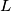$L$ and the circumcircle of triangle$ABC$ again at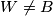$W \neq B$. Let$K$ be the perpendicular projection of$L$ onto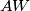$AW$. The circumcircle of triangle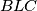$BLC$ intersects line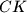$CK$ again at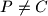$P \neq C$. Lines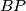$BP$ and$AW$ meet at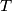$T$. Prove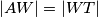$|AW| = |WT|$.

Izvor: Srednjoeuropska matematička olimpijada 2018, ekipno natjecanje, problem 6